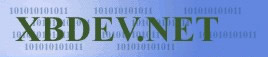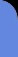Saturday May 25, 2019
 home | about | contact | Donations

 p {margin-bottom:0; margin-top:0} Optimising Your C by bkenwright@xbdev.net   Everyone when learning C or even C++, should know the basics of optimising your code... as no matter how fast your CPU gets, you always want to squeeze more and more out of it....  Make your CPU really perform for you.  So we'll go through some common optimisation techniques that would be useful to just know...or maybe add to some of your code...     Lightning Fast Multiplication and Divide   Now when someone first said to me, how can you improve this line of code: int i=2; i = i*4;   Looking at it you think, its i*4....its a single line...it can't possibly be improved any more...can it?  Well this is where your secret knowledge of binary arithmetic comes in handy, as a binary shift left (<<), or shift right (>>) uses less CPU time than an arithmetic multiplication. Shifting left (<<) is multiplying by the power of 2, and shifting right (>>) is dividing by the power of 2....   Looking at the powers of 2.. 2 4 8 16 32 64 128 258 ....   So for our little example above, we have:     i = i*4; and we change it to:     i = i<<2;   Shifting it once, is the same as multiplying it by 2, shifting it two bits, is the same as multiplying it by 4 etc.   Lets use a real world example... now in the days of dos, or in console game programming such as GBA or XBOX possibly, you can access memory directly, and that case memory is seen as a single array of pixels.  And to set a pixel at (x, y) you need to do this: screen[y*480 + x] = colour;   But 480 isn't a power of 2?....Well this is where the magic comes in.... we can break 480 into 2 numbers,   512-32 = 480 y*(512-32) is the same as y*(480) multiply out gives: y*512 - y*32   And using our otimisation secret we get: (y<<9) - (y<<5)   So putting it all together gives: screen[(y<<9) - (y<<5) + x] = colour;   As remember binary shifts are extremely simple operations, and hence extremely fast...faster then the multiplication operation.     Loops.... Not inside the loop... One of the first things you need to remember with loops, is try and not put things inside the loop that you don't have to.... a good and a bad example will help demonstrate this:   Bad example: // bad loop example for(i=0; i<10; i++) {       int j = 2; // putting declarations in the loop when you don't have to       aa[i] = j; }   Better example: // better loop example int j = 2; for(i=0; i<10; i++) {       aa[i] = j; }     Of course we could put aa[i]=2 etc in the loop...but I was just demonstrating, that you should try and not put declarations or anything in the loop if you can avoid it.   Loop unrolling.. Now taking this next loop as an example of how unrolling our code, can improve its speed //simple loop int indx=0; for(i=0; i<=40; i++) {       aa[indx++].value = true; }   Using the wonderful trick of unrolling, we can improve the speed of our code. //unrolling the loop to improve speed int indx=0; for(i=0; i<=40; i+=2) {       aa[indx++].value = true;       aa[indx++].value = true; }   Why is this faster?... as each time around the loop we do a test to see if its finished, but with our improved code, where only doing it half as many times.  As the code would consist of a conditional jump and a jump condition.     Loop flipping.. At first this might seem a bit tricky to understand, but its to do with how the cpu does loops...   // using loop without-flipping int idx=0; for(i=0; i<80; i+=2) {       aa[indx++].value = true;       aa[indx++].value = true; }   // using loop with-flipping int idx=0; i=0; do {       aa[indx++].value = true;       aa[indx++].value = true;       i+=2; }while(i<80);   Flipping as we've done above, gets rid of the initial conditional jump at the very start of our loop (first time), and gets ride of one of those jump instructions from inside the loop. We only have one conditional jump instruction at the end.   With flipping and unrolling we've improved our execution speed!   NOTE: On a 486 upwards... an ADD costs one cycle, while an IMUL costs between 13 and 42 cycles!.  Thats worth noting :)   Further Loop Unrolling   [Under Construction]     Rounding Numbers the Fast Way A nice example of where you may want to round a number, is when maybe you want to have your graphics snap to grid?..hmmm?? This speed boost, will only work with numbers that are a power of 2 as with most optimisation tricks... We do this by AND'ing our number with a bit mask.   An example is always the best way I think... If you wanted to round a number to the nearest value of 4, you AND the number with 0xFC.  In binary this looks like 1111 1100.    Modulus %..speed up Well this optimisation trick, only works on modulus numbers that are a power of 2, and is relatively easy to implement. How it works, is you take the modulus number and subtract 1 from it, you then AND it with your number... e.g. 28 % 8 = 4 Is the same as: 28 & (8-1) = 4   Again it might seem a bit worrying how this work, but if you look at it at the binary level...it soon starts to fall into place.  For example, 8-1 gives 7, and the binary of 7 is111. So 28 -> 11100 binary 7   -> 00111 binary (e.g. 8-1)       & 00100  -> 4   Using #define to optimise your code This is a sweet optimisation trick, as each time you call a function its costing you a small amount of processor time...even if its small.  But if your calling this function from a loop, or the function is very small then why not use the #define statement.  This will put your code inline and uses the pre-processor. example:   #define fixetoint(x) ((x) << 8 )     Register Variables ...eax...ebx... There is a keyword in C called "register" which informs the compiler to try and have this variable use one of the internal CPU registers and not a a memory location.  And as you should know, nothings faster than the CPU registers...so you would do you code like this:   register int i;   But remember if you over use the register keyword it can also slow your code down!  But used wisely can give a 10-15% speed improvement :)   Sweet Tricks Usually with experience, and of course time, you learn all sorts of sneaky tricks that you can put into your code.  This is where we go over some of these here. Lets say you want to swap two variables.... int a and int b.  How would you go about this?  Well the traditional way would be: int a = 2; int b = 3; int temp;   temp = a; a = b; b = temp;   But this requires the use of another register/memory location called temp... How would we do it without temp? Here is the answer:   #define SWAP(a, b) \             a ^= b;  \             b ^= a;  \             a ^= b;   Where of course, ^ is exclusive OR, and a^=b would be the same as a=a^b;   And for those that are a bit sceptical, we'll do a demo with some simple binary :- a = 11 b = 10   a ^= b   =>  a=01 b ^= a   =>  b=11 a ^= b   =>  a=10   After the operation:   a=10 b=11   Almost like magic :)     A very similar method, to the above one for swapping... is as shown: #define SWAP(a, b) \                 x = x-y;  \     // (comment: would be the difference)                 y = y+x; \     // (comment: alter y depending on the difference)                 x = y-x;        // (comment: alter x by the difference to know get y) Of course, I prefair the XOR method myself.... but I thought I'd give it a quick mention, as I remember seeing it once... and its always worth knowing :)     Quick Division By 4 Sometimes it important to know if a number is exactly divisible by 4...especially with 32 bit machines.... a quick way to do this, without resorting to modulus is to use a bit of binary arithmetic:   UINT i;   if( i & 3 ) // if false, then the number will divide exactly into 4   Lets look at the last 2 bits to see what they mean:   00 - 0 01 - 1 10 - 2 11 - 3   5   = 0000 0101   //   5/4=1.25 8   = 0000 1000   //   8/4=2 50 = 0011 0010   //   50/4=12.5 60 = 0011 1100   //   60/4=14   Note: I mention it here...but the fact of the matter, if you look at the simplification of the modulus operation (%) earlier...you'll notice that its the same.     [More To Be Added] "inline" keyword
 Visitor: 9534626  { 209.237.238.175 } Copyright (c) 2002-2017 xbdev.net - All rights reserved. Designated tutorial and software are the property of their respective owners.